Practice the AP 9th Class Physical Science Bits with Answers Chapter 6 Chemical Reactions and Equations on a regular basis so that you can attempt exams with utmost confidence.

## AP State Syllabus 9th Class Physical Science Bits 6th Lesson Chemical Reactions and Equations with Answers

Question 1.
C6H12O6 → C2H5OH + CO2 is ………………. chemical reaction.
A) combination
B) decomposition
C) displacement
D) double decomposition
B) decomposition

Question 2.
BaCl2 + Na2SO4 → BaSO4 + 2 NaCl represents ……………….
i) Decomposition reaction
ii) Displacement reaction
iii) Precipitation reaction
iv) Double displacement reaction
A) Only (i)
B) (ii) & (iii)
C) (iii) & (iv)
D) Only (iv)
C) (iii) & (iv)Question 3.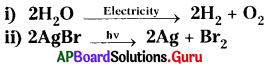The above reactions are examples for …………………
A) Chemical combination
B) Chemical decomposition
C) Chemical displacement
D) Double displacement
B) Chemical decomposition

Question 4.
The compound which emits Crimson Red colour flame is ………………..
A) Strontium chloride.
B) Cupric chloride.
C) Calcium chloride.
D) Sodium chloride.
A) Strontium chloride.

Question 5.
The iron nail dipped in Copper Sulphate solution becomes brown and the blue colour of the Copper Sulphate solution fades. Which type of reaction is this ?
A) Chemical combination
B) Chemical decomposition
C) Double decomposition
D) Displacement
D) Displacement

Question 6.
2PbO + C → 2Pb + CO2 is an example of
A) Oxidation reaction
B) Reduction reaction
C) Redox reaction
D) Corrosion reaction
C) Redox reaction

Question 7.
Avogadro’s number among the following is
A) 6.02 × 1023
B) 6.02 × 1032
C) 6.02 × 1033
D) 6.02 × 1022
A) 6.02 × 1023

Question 8.
Burning of magnesium crackers is a reaction of ………………….
A) reduction
B) cracking
C) oxidation
D) galvanizing
C) oxidation

Question 9.
Zn + 2 HCl → ZnCl2 + H2 is an example for …………………..
A) Chemical combination
B) Chemical decomposition
C) Chemical displacement
D) Chemical double displacement
C) Chemical displacementQuestion 10.
The reaction that takes place when quicklime is added to water is ………………..
A) displacement reaction
B) gas liberating reaction
C) heat liberating reaction
D) combustion reaction
C) heat liberating reaction

Question 11.
Number of moles of Oxygen needed to produce 4 moles of water on reacting with 4 moles of Hydrogen gas is ………………….
A) 1 mole
B) 2 moles
C) 3 moles
D) 4 moles
B) 2 moles

Question 12.
The balanced chemical equation of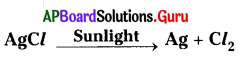is …………………….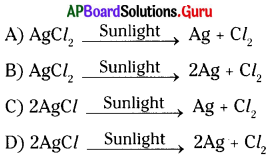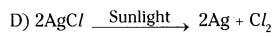Question 13.
The balanced chemical equation, among the following, is …………………..
A) NaOH + Zn → NaZnO2 + H2
B) 2NaOH + Zn → Na2ZnO2 + H2
C) 2NaOH + 2Zn → 2NaZnO2 + H2
D) NaOH + 2Zn → NaZn2O2 + H2
B) 2NaOH + Zn → Na2ZnO2 + H2

Question 14.
An oxidation reaction involves addition of ……………………
A) oxygen only
B) hydrogen only
C) oxygen or removal of hydrogen
D) hydrogen or removal of oxygen
C) oxygen or removal of hydrogen

Question 15.
An arrow pointing downwards in a chemical reaction shows ……………….
A) Evolution of gas
B) Formation of precipitate
C) Oxidation
D) Displacement
B) Formation of precipitate

Question 16.
CaO reacts with water to form ………………….
A) CaCO3
B) Ca(OH)2
C) CaCl2
D) Ca(NO3)2
B) Ca(OH)2Question 17.
Ammonia is formed by the reaction of gases …………………
A) H2 and O2
B) N2 and O2
C) N2 and H2
D) H2 and He
C) N2 and H2

Question 18.
The reaction Zn + H2SO4 → ZnSO4 + H2 represents ………………. reaction.
A) combination
B) double displacement
C) displacement
D) decomposition
C) displacement

Question 19.
A solution of potassium iodide reacts with lead nitrate to give …………………
A) KNO3
B) PbI2
C) A and B
D) PbO
C) A and B

Question 20.
The reaction of lead nitrate with dil. HCl represents ……………..
A) combination
B) decomposition
C) displacement
D) double decomposition
D) double decomposition

Question 21.
A solution of sodium carbonate reacts with Ca(OH)2 to give ……………….
A) CaCO3
B) NaOH
C) Both A and B
D) CaO
C) Both A and B

Question 22.
Which reaction is a reversible reaction ?
A) S + O2 → SO2
B) PCl5 ⇌ PCl3 + Cl2
C) 2 Na + Cl2 → 2 NaCl
D) CaC03 → CaO + CO2
B) PCl5 ⇌ PCl3 + Cl2

Question 23.
The reaction between Br2 and KI is a …………………. reaction.
A) combination
B) decomposition
C) displacement
D) double decomposition
C) displacementQuestion 24.
Due to which of the following enzymes, the surface of the fruit changes the colour on cutting ?
A) amylase
B) tyrosinase
C) lipase
D) peptidase
B) tyrosinase

Question 25.
Which of the following vitamins prevents the spoilage of food ?
A) A
B) K
C) C
D) D
C) C

Question 26.
NaCl(aq) + AgNO3(aq) → AgCl↓(s) + NaNO3(aq)
Which type of reaction does the above equation show ?
A) combination reaction
B) decomposition reaction
C) displacement reaction
D) double displacement reaction
D) double displacement reaction

Question 27.
To prevent which of the following reactions nitrogen gas is filled in chips flush bags ?
A) oxidation
B) reduction
C) corrosion
D) combustion
A) oxidation

Question 28.
Which of the following is formed, when magnesium burns in air ?
A) White coloured magnesium oxide
B) Black coloured magnesium oxide
C) Yellow coloured magnesium oxide
D) Red coloured magnesium oxide
A) White coloured magnesium oxide

Question 29.
CuO + H2 → Cu + H2O. In this reaction, which one is reduced ?
A) CuO
B) H2
C) H2O
D) CU
A) CuO

Question 30.
When corrosion of silver occurs black coating appears. The black coating indicates ……………….
A) silver sulphate
B) silver oxide
C) sliver sulphide
D) sliver chloride
C) sliver sulphideQuestion 31.
CaO + H2O → Ca(OH)2 → + Q (heat energy) The above reaction is an example of ……………….
A) combination
B) decomposition
C) displacement
D) double displacement
A) combination

Question 32.
Zn + 2HCl → ZnCl2 + H2
The above reaction is an example of ………………..
A) combination
B) decomposition
C) displacement
D) double displacement
C) displacement

Question 33.
When some metals are exposed to moisture, acids, etc. they tarnish due to formation of respective metal oxide on their surface. This process is called
A) reduction
B) combustion
C) corrosion
D) rancidity
C) corrosion

Question 34.
Rising of dough with yeast depends on ……………… of sugars to carbon dioxide and water.
A) rancidity
B) corrosion
C) reduction
D) oxidation
D) oxidation

Question 35.
By using which of the following one, we can calculate the number of molecules and atoms of different substances from the equation
A) Molar mass & unified
C) Both A and B
D) A Only
D) A Only

Question 36.
Which of the following one is hard and does not rust ?
A) iron
B) copper
C) stainless steel
D) none of these
C) stainless steel

Question 37.
To slow down which of the following, we keep the food in air tight containers ?
A) corrosion
B) oxidation
C) reduction
D) combustion
B) oxidationQuestion 38.
Corrosion is a / an …………………… reaction.
A) Oxidation
B) Reduction
C) Redox
D) None of these
A) Oxidation

Question 39.
Precipitate in a reaction is indicated by which arrow mark ?
A) →
B) ↑
C) ↓
D) ←
C) ↓

Question 40.
Calcium hydroxide is a ………………….. solution.
A) Acidic
B) Basic
C) Neutral
D) Amphoteric
B) Basic

Question 41.
A balanced equation contains
A) Equal number of moles of reactants and products
B) Equal number of molecules of reactants and products
C) Equal number of atoms of different elements on reactant side and product side
D) All the above
C) Equal number of atoms of different elements on reactant side and product side

Question 42.
Unbalanced equation is called
A) Basic equation
B) Skeleton equation
C) Stoichiometric equation
D) Fundamental equation
B) Skeleton equation

Question 43.
A chemical equation which contains the same number of atoms of different elements on reactant side and product side is
A) Skeleton equation
B) Balanced equation
C) Unbalanced equation
D) Any one of the above
B) Balanced equation

Question 44.
Metals displace hydrogen gas from dilute acids. This is an example for
A) combination reaction
B) decomposition reaction
C) displacement reaction
D) double decomposition reaction
C) displacement reactionQuestion 45.
When iron nail dipped in CuSO4 the nail becomes brown due to
A) deposition of copper on iron
B) dissolution of iron
C) reduction of iron
D) oxidation of Cu
A) deposition of copper on iron

Question 46.
Which of the following combinations is wrong ?
A) 2N2O → 2N2+ O2 – decomposition
B) Ca + 2H2O → Ca(OH)2 – combination
C) Zn + 2 HCl → ZnCl2 + H2 – double decomposition
D) Zn + H2SO4 → ZnSO4 + H2 – displacement
C) Zn + 2 HCl → ZnCl2 + H2 – double decomposition

Question 47.
Why does moist Cl2 act as bleaching agent ?
A) In presence of water, Cl2 gives nascent hydrogen.
B) In presence of water, Cl2 gives nascent oxygen.
C) Moist Cl2 imparts colour to the substance.
D) Moist chlorine liberates nascent chlorine.
B) In presence of water, Cl2 gives nascent oxygen.

Question 48.
The reagent used to remove the colour of the matter is called
A) chlorinating reagent
B) oxidising agent
C) bleaching agent
D) reducing agent
C) bleaching agent

Question 49.
Match the following.
Oxide – Colour
A) MgO a) White
B) PbO b) Yellow
C) CuO c) Black
D) CaO d) Colourless
A) 1 – a, 2 – b, 3 – c, 4 – d
B) 1 – a, 2 – b, 3 – d, 4 – c
C) 1 – d, 2 – c, 3 – b, 4 – a
D) 1 – d, 2 – c, 3 – a, 4 – b
A) 1 – a, 2 – b, 3 – c, 4 – d

Question 50.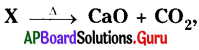Ca(OH)2 + CO2 → Y + H2O
The X and Y are
A) CaCO3, Ca(OH)2
B) Ca(HCO3)2, CaCO3
C) CaCO3, Ca(HCO3)2
D) CaCO3, CaCO3
D) CaCO3, CaCO3

Question 51.
Which of the following statements is wrong ?
A) Conversion of milk into curd is a chemical change.
B) Addition of water to quick lime liberates heat energy.
C) Addition of aqueous Na2SO4 to aqueous BaCl2 forms clear solution.
D) Calcium oxide produces colourless solution when dissolved in water.
C) Addition of aqueous Na2SO4 to aqueous BaCl2 forms clear solution.

Question 52.
Match the following :

 a) combination reaction A) 2 AgNO3 + Na2CrO4 → Ag2CrO4 + 2NaNO3 b) decomposition reaction B) 2NH3 → N2 + 3H2 c) displacement reaction C) C2H4 + H2O → C2H6O d) double displacement reaction D) Fe2O3 + 3CO → 2Fe + 3CO2

A) 1 – a, 2 – b, 3 – c, 4 – d
B) 1 – d, 2 – b, 3 – a, 4 – c
C) 1 – b, 2 – d, 3 – c, 4 – a
D) 1 – d, 2 – c, 3 – b, 4 – a
B) 1 – d, 2 – b, 3 – a, 4 – cQuestion 53.
Rancidity means
A) Improve the quality of food
B) Improve the preservation of food
C) Spoilage of food by oxidation
D) Any one of the above
C) Spoilage of food by oxidation

Question 54.
The number of molecules present in 1g of hydrogen
A) 6 × 1023
B) 3 × 1023
C) 1 × 1023
D) 2 × 1023
B) 3 × 1023

Question 55.
Which of the following is not an exothermic reaction?
A) C + O2 → CO2
B) CaO + H2O → Ca(OH)2
C) N2 + O2 → 2NO
D) CH4 + 2O2 → CO2 + 2H2O
C) N2 + O2 → 2NO

Question 56.
Which of the following statements is / are true?
A) Molecular mass is expressed in unified mass (U).
B) 22.4 l of any gas at STP contains 6.023 × 1023 molecules.
C) 28 g of N2 at STP occupies 22.4 litres of volume.
D) All are correct.
D) All are correct.

Question 57.
Heating of which of the following compounds gives acidic and basic oxide ?
A) Pb(NO3)2
B) AgCl
C) CaCO3
D) All the above
C) CaCO3

Question 58.
Choose double displacement reaction ………………….
A) X + YZ → XZ + Y
B) X + Y → XY
C) XYZ → XY + Z
D) XY + AB → AX + BY
D) XY + AB → AX + BY

Question 59.
Which is not true in a balanced equation ?
A) Number of atoms of different elements on both sides are equal.
B) Mass of both sides are equal.
C) Number of charged ions on both sides are equal.
D) Total charges on both sides are equal.
C) Number of charged ions on both sides are equal.Question 60.
Which of the following is an example of displacement reaction ?
A) N2 + 3H2 → 2NH3
B) 2KClO3 → 2KCl + 3O2
C) Zn + 2HCl → ZnCl2 + H2
D) 2H2 → O2 → 2H2O
C) Zn + 2HCl → ZnCl2 + H2

Question 61.
Which is true about oxidation ?
A) It involves loss of electrons.
B) It involves addition of oxygen.
C) It involves removal of hydrogen.
D) All are true.
D) All are true.

Question 62.
In the equation CuO + H2 → Cu + H2O, the substance reduced is ………………
A) H2
B) CU
C) CuO
D) H2O
C) CuO

Question 63.
Which of the statements about the reaction given below are incorrect ?
2 PbO(s) + C(s) → 2Pb(s) + CO2(g)
1) Lead is getting reduced. ( )
2) CO2 is getting oxidised.
3) C is getting oxidised.
4) Pbo is getting reduced.
A) 1 and 2
B) 1 and 3
C) 1, 2, and 3
D) all
B) 1 and 3

Question 64.
Silver chloride is mainly used in ……………….
A) In cooking special items
B) In black and white photography
C) A’ and ‘B’
D) In coloured photography
B) In black and white photography

Question 65.
Which of the following is not a redox reaction ?
A) AgNO3 + NaCl → AgCl + NaNO3
B) CuO + H2 → Cu + H2O
C) Mg + Cl2 → MgCl2
D) MnO2 + 4 HCl → MnCl2 + 2H2O + 2Cl2
A) AgNO3 + NaCl → AgCl + NaNO3

Question 66.
In which of the following, heat energy will be evolved ?
A) Burning of L.P.G.
B) Electrolysis of water
C) Dissolution of ammonium chloride in water
D) Decomposition of silver bromide in the presence of sunlight
A) Burning of L.P.G.

Question 67.
When copper vessels are kept over a period of time, these get coated with a green surface. This is due to ………………
1) Oxidation of copper
2) Corrosion of copper
3) Reduction of copper
4) Hydrolysis of copper
A) 1, 2
B) 3, 4
C) 1, 4
D) 2, 4
A) 1, 2Question 68.
The chemical formula of ferric oxide is ………………..
A) Fe2O
B) Fe2O3
C) FeO
D) FeO2
B) Fe2O3

Question 69.
Which information is not conveyed by a balanced chemical equation ?
A) Symbols and formulae of all the substances involved in a particular reaction
B) Number of atoms / molecules of the reactants and products involved
C) Physical states of reactants and products
D) Whether a particular reaction is actually possible or not
D) Whether a particular reaction is actually possible or not

Question 70.
Which of the following are combination reactions ………………….
1) 2KClO4 → 2KCl + 4O2
2) MgO + H2O → Mg(OH)2
3) 4Al + 3O2 → 2Al2O3
4) Zn + FeSO4 → ZnSO4 + Fe
A) 2, 4
B) 1, 3
C) 3
D) 2, 3
D) 2, 3

Question 71.
Which among the following are double displacement reactions ?
1) Pb + CuCl2 → PbCl2 + Cu
2) Na2SO4 + BaCl2 → BaSO4 + 2NaCl
3) C + O2 = CO2
4) CH4 + 2O2 → CO2 + 2H2O
A) 1, 2
B) 2
C) 1, 4
D) 2, 4
B) 2

Question 72.
Which of the following is not an exothermic reaction ? …………………
1) C + O2 → CO2
2) N2 + O2 → 2NO
3) CaCO3 → CaO + CO2
4) HCl(aq) + NaOH(aq) → NaCl(aq) + H2O(l)
A) 2
B) 2, 3
C) 1
D) 1, 4
B) 2, 3

Question 73.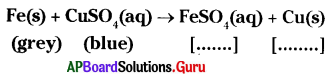A) brown, grey
B) blue, brown
C) green, brown
D) blue, grey
C) green, brown

Question 74.
What is the wrong in the following equation ?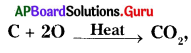it can be corrected as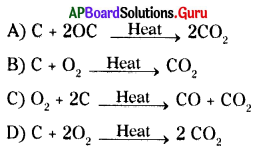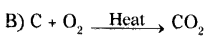Question 75.
The following reaction is an example of a …………………
4NH3(g) + 5O2(aq) → 4NO(g) + 6H2O(l)
1) Displacement reaction
2) Combination reaction
3) Redox reaction
4) Neutralisation reaction
A) 2, 3
B) 3, 4
C) 1, 3
D) 1, 4
A) 2, 3

Question 76.
In the reaction :
Zn + FeSO4 → ZnSO4 + Fe
A) Zn and Fe are both oxidised
B) Fe gets oxidised
C) Zn gets oxidised
D) Zn is an oxidising agent
C) Zn gets oxidised

Question 77.
Which of the following are endothermic processes ? …………………….
1) Dilution of sulphuric acid
2) Sublimation of dry ice
3) Condensation of water vapour
4) Evaporation of water
A) 3
B) 1, 3
C) 2
D) 2, 4
D) 2, 4

Question 78.
Chemical reactions which occur in presence of sunlight are called ………………. reactions.
A) Thermal
B) Electrolysis
C) Photo chemical
D) Light
C) Photo chemical

Question 79.
Barium chloride on reacting with ammonium sulphate forms barium sulphate and ammonium chloride. Which of the following correctly represents the type of reaction involved ?
1) displacement reaction
2) precipitation reaction
3) combination reaction
4) double displacement reaction
A) 4
B) 1
C) 2
D) 2, 4
D) 2, 4

Question 80.
Which of the following are exothermic processes ?
1) Reaction of water with quick lime
2) Dilution of an acid
3) Evaporation of water
4) Sublimation of camphor (crystals)
A) 1, 2
B) 3, 4
C) 2, 3
D) 1, 4
A) 1, 2

Question 81.
Which of the following is not a balanced equation ?
A) Fe + Cl2 → FeCl3
B) Zn + S → ZnS
C) Mg + CuSO4 → MgSO4 + Cu
D) NaOH + HCl → NaCl + H2O
A) Fe + Cl2 → FeCl3Question 82.
Assertion (A) :
CaO + H2O → Ca(OH)2 is a chemical change.
Reason (R) : The original substance does not loss their characteristic properties in chemical change.
A) Both ‘A’ and ‘R’ are correct and ‘R’ supports ‘A’.
B) Both ‘A’ and ‘R’ are correct but ‘R’ does not support ‘A’.
C) ‘A’ is correct but ‘R’ is incorrect.
D) Both ‘A’ and ‘R’ are incorrect.
C) ‘A’ is correct but ‘R’ is incorrect.

Question 83.
Assertion (A) : The number of atoms of each element before and after reaction must be the same.
Reason (R) : Law of conservation of mass is followed by every chemical reaction.
A) Both ‘A’ and ‘R’ are correct and ‘A’ is supported by ‘R’.
B) Both ‘A’ and ‘R’ are correct, but ‘A’ is not supported by ‘R’.
C) ‘A’ is correct, but ‘R’ is incorrect.
D) ‘A’ is incorrect, but ‘R’ is correct.
A) Both ‘A’ and ‘R’ are correct and ‘A’ is supported by ‘R’.

Question 84.
Assertion (A) : All the chemical equations must be balanced.
Reason (R) : Atoms are either created or destroyed in chemical reaction.
A) Both ‘A’ and ‘R’ are correct and ‘A’ is supported by ‘R’.
B) Both ‘A’ and ‘R’ are correct, but ‘A’ is not supported by ‘R’.
C) ‘A’ is correct, but ‘R’ is incorrect.
D) ‘A’ is incorrect, but ‘R’ is correct.
C) ‘A’ is correct, but ‘R’ is incorrect.

Question 85.
Assertion (A) :
2C3H8 + 10O2 → 6CO2 + 8H2O is not a balanced equation.
Reason (R) : The coefficients are not the smallest whole numbers.
A) Both ‘A’ and ‘R’ are correct and ‘A’ is supported by ‘R’.
B) Both ‘A’ and ‘R’ are correct, but ‘A’ is not supported by ‘R’.
C) ‘A’ is correct, but ‘R’ is incorrect.
D) ‘A’ is incorrect, but ‘R’ is correct.
A) Both ‘A’ and ‘R’ are correct and ‘A’ is supported by ‘R’.

Question 86.
Assertion (A) : All the decomposition reactions are endothermic.
Reason (R) : Decomposition reactions require energy in the form of heat, light or electricity.
A) Both ‘A’ and ‘R’ are correct and ‘A’ is supported by ‘R’.
B) Both ‘A’ and ‘R’ are correct, but ‘A’ is not supported by ‘R’
C) ‘A’ is correct, but ‘R’ is incorrect.
D) ‘A’ is incorrect, but ‘R’ is correct.
A) Both ‘A’ and ‘R’ are correct and ‘A’ is supported by ‘R’.

Question 87.
Assertion (A) : The element zinc has displaced hydrogen from hydrochloric acid.
Reason (R): Generally metals which are less active than hydrogen displaces it from an acid.
A) Both ‘A’ and ‘R’ are correct and ‘A’ is supported by ‘R’.
B) Both ‘A’ and ‘R’ are correct, but ‘A’ is not supported by ‘R’.
C) ‘A’ is correct, but ‘R’ is incorrect.
D) ‘A’ is incorrect, but ‘R’ is correct.
C) ‘A’ is correct, but ‘R’ is incorrect.Question 88.
Assertion (A) : Fe + CuSO4 → FeSO4 + Cu.
Reason (R) : Copper is more reactive than iron.
A) Both ‘A’ and ‘R’ are correct and ‘A’ is supported by ‘R’.
B) Both ‘A’ and ‘R’ are correct, but ‘A’ is not supported by ‘R’.
C) ‘A’ is correct, but ‘R’ is incorrect.
D) ‘A’ is incorrect, but ‘R’ is correct.
C) ‘A’ is correct, but ‘R’ is incorrect.

Question 89.
Assertion (A) : Oxidation take place in metals only.
Assertion (B) : Corrosion is an oxidation process.
A) A and B are correct.
B) A is correct, B is incorrect.
C) A is incorrect, B is correct.
D) Both A and B are incorrect.
C) A is incorrect, B is correct.

Question 90.
Assertion (A) : Chips packets are filled with Vitamin ‘C’ and Vitamin ‘E’.
Reason (R) : Vitamin ‘C’ and Vitamin ‘E’ prevents oxidation.
A) ‘A’, ‘R’ are correct, A is supported by ‘R’.
B) ‘A’, ‘R’ are correct, A is not supported by ‘R’.
C) ‘A’ is correct, ‘R’ is incorrect.
D) ‘A’ is incorrect, ‘R’ is correct.
D) ‘A’ is incorrect, ‘R’ is correct.

Question 91.
Select the correct option given below.
a) Chemical combination is always endothermic.
b) Chemical decomposition is always exothermic.
A) ‘a’ and ‘b’ are correct.
B) ‘a’ is correct and ‘b’ is incorrect.
C) ‘a’ is incorrect and ‘b’ is correct.
D) both ‘a’ and ‘b’ are incorrect.
D) both ‘a’ and ‘b’ are incorrect.

Question 92.
Which of the following is true in a completed chemical reaction ?
A) The total mass of the products > total mass of the reactants.
B) The total mass of the products < total mass of the reactants.
C) The total mass of the products = total mass of the reactants.
D) The total mass of the products × total mass of the reactants = 0.
C) The total mass of the products = total mass of the reactants.

Question 93.
x KClO3 → y KCl + z O2. The respective values of x, y, z are ………………….
A) 1, 2, 3
B) 3, 3, 2
C) 2, 2, 3
D) 2, 2, 2
C) 2, 2, 3

Question 94.
A neutralization reaction is a ………………. reaction.
A) decomposition
B) displacement
C) combination
D) double displacement
D) double displacementQuestion 95.
In which of the following reactions is there only one reactant ?
A) combination
B) decomposition
C) displacement
D) double displacement
A) combination

Question 96.
In a decomposition reaction of CuCO3 which one is formed ?
A) CO2
B) O2
C) H2
D) N2
A) CO2

Question 97.
The gas liberated when zinc carbonate decomposes is …………………
A) Cl2
B) CO2
C) O2
D) NO2
B) CO2

Question 98.
Zinc displaces the following gas from sulphuric acid ………………
A) O2
B) CO2
C) NO2
D) H2
D) H2

Question 99.
Which of the following gases puts off the matchstick when calcium carbonate is heated ?
A) H2
B) O2
C) CO2
D) NO2
C) CO2

Question 100.
Which of the following gases indicates the brown fumes liberating in the boiling tube, when lead nitrate is heated?
A) H2
B) O2
C) CO2
D) NO2
D) NO2

Question 101.
Which of the following precipitates is formed, on mixing lead nitrate [Pb(NO3)2] with potassium iodide [KI]?
A) Brown coloured PbI2
B) Green coloured PbI2
C) Blue coloured PbI2
D) Yellow coloured PbI2
D) Yellow coloured PbI2Question 102.
When corrosion of copper occurs, green coating appears on copper. The green coating indicates
A) Cu
B) Cu2O
C) CuO
D) CuO2
C) CuO

Question 103.
A substance is in light yellow colour. If we put it in sunlight, it changes into grey colour. What is the substance ?
B) Potassium iodide
C) Silver bromide
D) HCl
C) Silver bromide

Question 104.
x H2 + y O2 > z H2O. The values of
x, y, z are
A) x = 1, y = 1, z = 1
B) x = 2, y = 1, z = 2
C) x = 2, y = 2, z = 2
D) x = 2, y = 1, z = 1
B) x = 2, y = 1, z = 2

Question 105.
Which of the following is not a double decomposition reaction ?
A) Pb(NO3)2 + 2 KI → PbI2 + 2KNO3
B) NaCl + AgNO3 → AgCl + NaNO3
C) Na2SO4 + BaCl2 → BaSO4 + 2NaCl
D) Fe + CuSO4 → FeSO4 + Cu
D) Fe + CuSO4 → FeSO4 + Cu

Question 106.
Why are food materials packed in air tight containers ?
A) To preserve the nutrients
B) To prevent the spoilage by oxidation
C) To prevent the spoilage by reduction
D) To improve the taste
B) To prevent the spoilage by oxidation

Question 107.
Heating of Pb(NO3)2 gives two gases ‘X’ and ‘Y’ The colour of X and Y is
A) X = Colourless, Y = Colourless
B) X = Red, Y = Brown
C) X = Brown, Y = Colourless
D) X = Green, Y = Yellow
C) X = Brown, Y = ColourlessQuestion 108.
In electrolysis of water experiment, the ratio of volumes of oxygen and hydrogen gases evolved is ………………….
A) 1 : 2
B) 2 : 1
C) 1 : 1
D) 3 : 1
A) 1 : 2

Question 109.
The process of preparing slaked lime by adding water to quicklime is this type of chemical reaction.
A) Decomposition reaction
B) Exothermic reaction
C) Endothermic reaction
D) Displacement reaction
B) Exothermic reaction

Question 110.
If the gas liberated in an experiment allows the burning splinter to continue burning more brightly in its presence, the gas is ………………….
A) oxygen
B) nitrogen
C) hydrogen
D) carbon dioxide
A) oxygen

Question 111.
Solutions of Copper sulphate, Iron sulphate and Sodium sulphate are marked as X, Y and Z respectively. Few pieces of Aluminium are added to each solution.
In which solutions, change will be observed ?
A) X and Y
B) Y and Z
C) X and Z
D) X, Y and Z
A) X and Y

Question 112.
When a small piece of zinc metal is added to a solution of copper sulphate and on heating the products formed are ………………
A) CuO
B) ZnSO4 + Cu
C) ZnO
D) H2
B) ZnSO4 + Cu

Question 113.
Which of the following precipitates is formed on mixing sodium sulphate with barium chloride solution?
A) White precipitate of barium sulphate
B) Black precipitate of barium sulphate
C) Yellow precipitate of barium sulphate
D) Red precipitate of barium sulphate
A) White precipitate of barium sulphate

Question 114.
Which of the following is the colour of hydrous copper sulphate (CuSO4) ?
A) Red
B) White
C) Yellow
D) Blue
D) BlueQuestion 115.
When dilute hydrochloric acid is added to iron filings
A) hydrogen gas and iron chloride are formed
B) chlorine gas and iron hydroxide are formed
C) no reaction takes place
D) iron salt and water are produced
A) hydrogen gas and iron chloride are formed

Question 116.
Which of the following metals fails to evolve hydrogen gas with dilute HCl? ………………….
A) Iron
B) Aluminium
C) Zinc
D) Copper
D) Copper

Question 117.
In the electrolysis of water, anode
A) has positive charge
B) is connected to negative terminal of battery
C) is neutral
D) has negative charge
A) has positive charge

Question 118.
A student takes about 2 ml ethanoic acid in a dry test tube and adds a pinch of sodium hydrogen carbonate to it. He reports the following observations
1) Immediately a colourless and odourless gas evolves with a brisk effervescence
2) The gas turns lime water to milky when passed through it.
3) The gas burns with an explosion when a burning splinter is brought near to it.
4) The gas extinguishes the burning splinter that is brought near to it
A) 1, 2, 3
B) 2, 3, 4
C) 3, 4, 1
D) 1, 2, 4
D) 1, 2, 4

Question 119.
Copper is more reactive than silver. This is explained as
Cu + AgNO3(aq) → CuO(NO3)2(aq) + 2Ag. It reveals
A) It displaces silver from silver nitrate thus giving shining appearance on top
B) It decomposes silver from silver nitrate giving no appearance change
C) Both A’ and ‘B’ sometimes
D) Not explained
A) It displaces silver from silver nitrate thus giving shining appearance on top

Question 120.
The correct observation made by the student after putting clean pieces of iron in the test tube containing zinc sulphate is as shown in figure :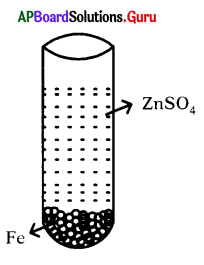A) Solution becomes colourless and zinc gets deposited on iron
B) Solution becomes green and zinc gets deposited on iron
C) Iron pieces get dissolved in the solution making it green
D) No reaction is observed
D) No reaction is observed

Question 121.
A student added dilute HCl to a test tube containing Zn granules and made following observations ?
1) The Zn surface became dull and black
2) A gas evolved which burnt with a pop sound
3) The solution remained colourless
A) 1, 2
B) 2, 3
C) 2, 3
D) all?
B) 2, 3Question 122.
Assertion (A) : Heat is released on reaction of water with CaO,
Reason (R) : It is an exothermic reaction.
A) Both (A), (R) are correct, Assertion supports Reason.
B) Both (A), (R) are correct, Assertion does not support Reason.
C) (A) is correct, (R) is wrong.
D) (A) is wrong, (R) is correct.
A) Both (A), (R) are correct, Assertion supports Reason.

Question 123.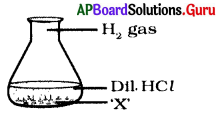‘X’ indicates
A) Salt
B) Metal
C) Non-metal
D) Base
B) Metal

Question 124.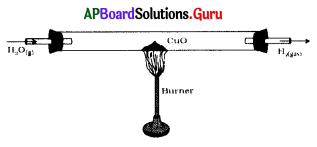Identify the wrong in the figure.
A) Chemicals used
B) Place of burner
C) Direction of gas
D) Position of the corks
C) Direction of gas

Question 125.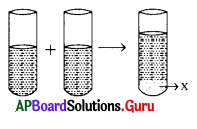Pb(NO3)2 KI
What does ‘X’ indicate ?
A) PbK
B) PbI2
C) PbKI(NO3)2
D) NO3Pb
B) PbI2

Question 126.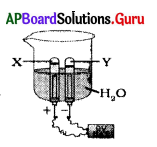Label ‘X’ and ‘Y’
A) O2, H2
B) Cl2, O2
C) H2, O2
D) O2, Cl2
A) O2, H2

Question 127.
A balanced chemical equation is appreciable because, it gives
A) mass – mass relationship
B) mass – volume relationship
C) volume – volume relationship
D) above all
D) above all

Question 128.
6CO2 + 6H2O → C6H12O6 + 6O2. This reaction is appreciable, because it gives us
A) sunlight
B) chlorophyll
C) glucose
D) heat
C) glucoseQuestion 129.
Vitamin ‘C’ and Vitamin ‘E’ are appreciable because, they can prevent
A) Rancidity
B) Alloying
C) Reduction
D) Above all
A) Rancidity

Question 130.
We should appreciate alloys, because they
A) do not corrode
B) have hardness
C) have strength
D) above all
D) above all

Question 131.
Which of the following is an oxidation process observed in daily life ?
A) Black coating on silver
B) Formation of green compound on copper
C) Fermentation
D) All the above
D) All the above

Question 132.
Chemically rust is
A) only ferric oxide
B) hydrated ferric oxide
C) hydrated cuprous oxide
D) hydrated ferrous oxide
B) hydrated ferric oxide

Question 133.
Identify methods for prevention of rusting from the following.
1) By applying oils and greases on surfaces
2) By paint coating on surfaces
3) By galvanisation
4) By keeping the iron articles in air
A) 1, 2
B) 1, 2, 3
C) 4
D) 2, 4
B) 1, 2, 3

Question 134.
Rancidity is ……………….. reaction.
A) Reduction
B) Oxidation
C) Redox
D) Double displacement
B) Oxidation

Question 135.
Stainless steel is an alloy of iron with elements like ………………
A) Cu, Ni, Cr
B) C, Ni, Cr
C) Fe, C, Cr
D) Fe, Ni, Cr
B) C, Ni, CrQuestion 136.
Which of the following gases can be used for storage of fresh sample of an oil for a long time ?
1) Carbon dioxide
2) Helium
3) Nitrogen
4) Oxygen
A) 1 or 4
B) 3 or 4
C) 2 or 3
D) 1 or 2
C) 2 or 3

Question 137.
Coating of thin layer of zinc on metal surface is called …………………..
A) Galvanizing
B) Zinc coating
C) Corrosion
D) Rusting
A) Galvanizing

Question 138.
Three beakers labelled as A, B and C each containing 25 ml of water were taken. A small amount of NaOH, an hydrous CuSO4, and NaCl were added to the beakers A, B and C respectively. It was observed that there was an increase in temperature of solution, contained in beakers A and B, where as in beaker C, the temperature of the
solutions falls. Which one of the following statements is / are correct ?
1) In beakers ‘A’ and ‘B’, exothermic process has occurred
2) In beakers ‘A’ and ‘B’, endothermic process has occurred
3) In beaker ‘C’, exothermic process has occurred
4) In beaker ‘C’, endothermic process has occurred
A) 2
B) 2, 3
C) 1, 4
D) 1
C) 1, 4

Question 139.
Quick lime reacts vigorously with water releasing a large amount of heat. Which type of reaction is this ?
1) Precipitation reaction
2) Combination reaction
3) Decomposition reaction
4) Exothermic reaction
A) 1, 2
B) 2, 3
C) 2, 4
D) 4